×#### Thank you for registering.

One of our academic counsellors will contact you within 1 working day.

Click to Chat

1800-1023-196

+91-120-4616500

CART 0

• 0

MY CART (5)

Use Coupon: CART20 and get 20% off on all online Study Material

ITEM
DETAILS
MRP
DISCOUNT
FINAL PRICE
Total Price: Rs.

There are no items in this cart.
Continue Shopping• Complete JEE Main/Advanced Course and Test Series
• OFFERED PRICE: Rs. 15,900
• View Details

```Chapter 6: Graphs of Trigonometric Functions – Exercise 6.3

Graphs of Trigonometric Functions – Exercise 6.3 – Q.1

We know thatWe have,Substituting these values in (i), we getThus we draw the graph of Y = cos 2X, adjust the maximum and minimum values to 1/2 and -1/2 and shift it by 1/2 up to get the required graph.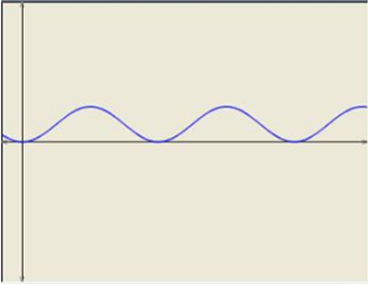Graphs of Trigonometric Functions – Exercise 6.3 – Q.2

We know thatWe have,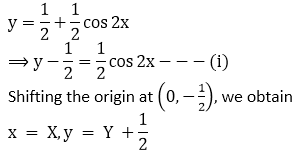Substituting these values in (i), we getThus we draw the graph of Y = cos 2X, adjust the maximum and minimum values to 1/2 and -1/2 and shift it by 1/2 up to get the required graph.Graphs of Trigonometric Functions – Exercise 6.3 – Q.3

We have,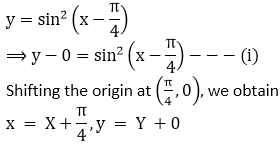Substituting these values in (i), we get

Y = sin2X.

Thus we draw the graph of Y = sin2 X, and shift it by π/4  to the rught to get the required graph.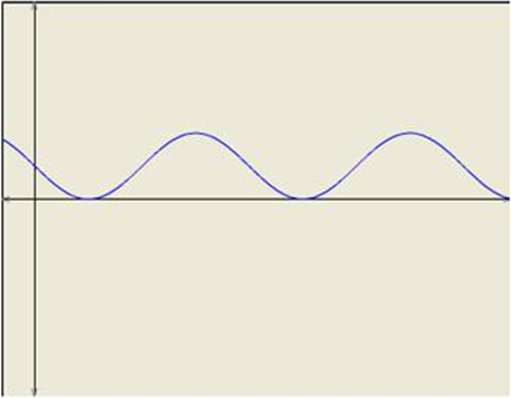Graphs of Trigonometric Functions – Exercise 6.3 – Q.4

To obtain the graph of y = tan 2x we first draw the graph of y = tan x in the intervaland then divide the x-coordinates of the points where it crosses x-axis by 2.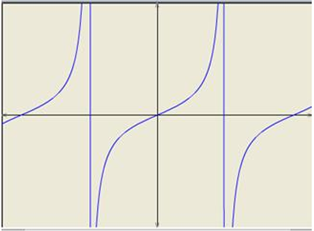Graphs of Trigonometric Functions – Exercise 6.3 – Q.5

To obtain the graph of y = tan 3x we first draw the graph of y = tan x in the intervaland then divide the x-coordinates of the points where it crosses x-axis by 3. We then stretch the graph vertically by a factor of 2.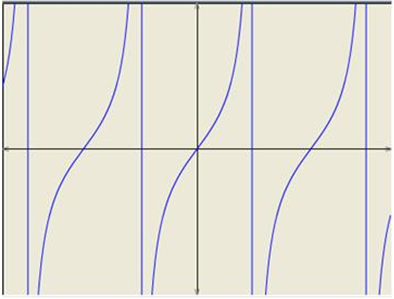Graphs of Trigonometric Functions – Exercise 6.3 – Q.6

To obtain the graph of y = 2 cot 2x we first draw the graph of y = cot x in the interval (0, π) and then divide the x-coordinates of the points where it crosses x-axis by 2. We then stretch the graph vertically by a factor of 2.Graphs of Trigonometric Functions – Exercise 6.3 – Q.7

We have,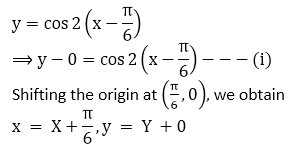Substituting these values in (i), we get

Y = cos 2X.

Thus we draw the graph of Y = cos 2X, and shift it by π/6 to the  rught to get the required graph.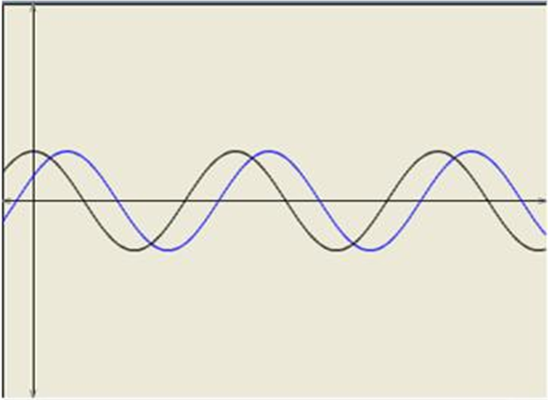Graphs of Trigonometric Functions – Exercise 6.3 – Q.8

We know thatWe have,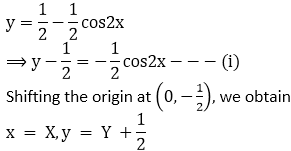Thus we draw the graph of Y = cos 2X, adjust the maximum and minimum values to 1/2 and -1/2 and shift it by 1/2 up to get the required graph.```### Course Features

• 728 Video Lectures
• Revision Notes
• Previous Year Papers
• Mind Map
• Study Planner
• NCERT Solutions
• Discussion Forum
• Test paper with Video Solution